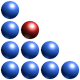# Complexity-based Control of Dynamic Systems

Complexity-based control is possible because complexity can be measured in real-time using specifically developed hardware. The concept is illustrated below, whereby a QCM block is used to drive the complexity of a given system to desired levels.Because high complexity can lead to instability and fragility, the goal of QCM control is to drive complexity below prescribed thresholds and far from critical complexity. It is well known that in proximity of critical complexity robustness drops increasing vulnerability. This fact may be used in a context of aggressive operations, whereby complexity of an enemy network can be increased so as to reduces its robustness (resilience). There are various ways of achieving this but they won’t be disclosed for obvious reasons. A simple example of a steady-state system in which complexity is increased is shown in the plot below. As complexity increases, robustness drops from 80% to around 60%. This may be enough to ‘soften’ enemy networks prior to an attack.However, a more popular goal of QCM control will certainly be to reduce complexity and not to increase it. High complexity (in any context) means lower governability. This is due to the fact that highly complex systems can function in many modes and go through (spontaneous) mode switching with very small amounts of energy (perturbations).

An example of complexity of a naturally decaying (uncontrolled) LTI (Linear Time Invariant) system is illustrated below. As the system dissipates energy, its complexity diminishes.Applying QCM-based gains accelerates energy dissipation and reduces complexity at a higher rate than in the uncontrolled case. This is shown below.The complexity-based gains are determined via a proprietary procedure which will not be described. However, for those who are familiar with LQG (Linear Quadratic Gaussian) control systems, one could minimize a cost function such as this:augmenting it with a quadratic complexity-specific component and solving a nonlinear differential Riccati matrix equation to yield the optimum feedback gains.

In alternative one could envisage a Hamiltonian-type of cost function in which Lagrange multipliers (costates) are used to incorporate a complexity component. Adopting the PMP (Pontryaghin’s Maximum Principle) can yield an optimal controller.

However, our QCM control utilizes a proprietary approach in which time-dependent complexity-based feedback gains need not be determined via costly optimization.

In a totally different context, a discrete QCM block can be programmed to react only to anomalies or complexity peaks such as in the case below, where a spike in complexity crosses a threshold.In this case, the QCM controller is dormant and kicks in only when a complexity peak is detected.

www.ontonix.com